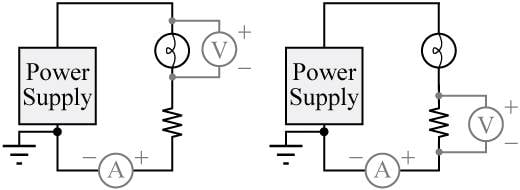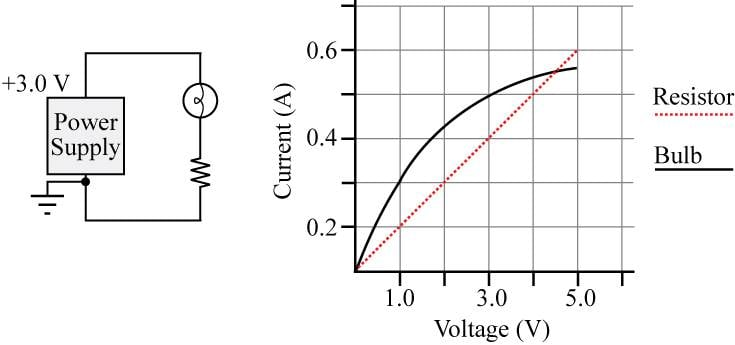# Variable Resistance across a Bulb

• Marshillboy
In summary, a student conducts an experiment with a light bulb and a resistor connected in series to a variable power supply. They measure the current and voltage across the bulb and resistor separately and plot the data on a graph. The question asks for the estimated current through the bulb when the power supply voltage is +3.0 V, which can be found by looking at a horizontal line on the graph where the two voltages add up to 3V. The answer is at a current of 0.3A, with 1V across the bulb and 2V across the resistor.

## Homework Statement

A light bulb and a resistor are connected in series to a variable power supply. A student first connects a voltmeter and an ammeter to measure the current through and the voltage across the bulb, as shown in the left circuit. The student varies the power supply voltage and records the readings on the meters.

The student then places the voltmeter across the resistor as shown in the right circuit, and repeats the experiment. She plots the data for both circuit elements in a single graph, as shown below.a)Suppose the power supply voltage is now set to +3.0 V. For this power supply voltage, is the absolute value of the voltage across the bulb greater than, less than, or equal to 3.0 V?

b)Use the graph to estimate the current through the bulb when the power supply voltage is +3.0 V.

V=IR

## The Attempt at a Solution

a) The two elements are in series, so I believe the voltage across the bulb would have to be less than 3V

b) I'm having trouble with this since the resistance of the bulb is varying.

You are absolutely correct... the resistance of the bulb is changing, it must be a bulb with a metal filament (but that has got nothing to do with this question!).
The most important thing here is to realize that bulb and resistor are in SERIES. That means that at all times the same CURRENT flows through both.
If you look at the graph and draw horizontal lines through the current values 0.2, 0.3, 0.4 and 0.5 then the voltage on the redline (the resistor) is always greater than the voltage on the curved black line (the bulb)
The most important thing about part (b) of the question is that it asks you to ESTIMATE the current in the circuit.
This usually means that you have to get the answer from a graph and there is not an exact answer... you have to show that you know what you are doing!
In this question you have 2 components connected in series so I hope you realize that the voltages across each one must add up to the battery voltage.
We are looking for 2 voltages (the red line and the black line) that add up to 3V for a particular current.
Look at a current of 0.2A... the voltages are (about 0.5 for the black line) and 1 for the red line... these do NOT add up to 3V
Look at current = 0.3A ...voltage for black line =1.0V and for the red line = 1.5V... ad up to 2.5V
Look at current = 0.4A ... volts for black line = nearly 1.5V and for the red line = 3.0V ...add up to 4.5V !
Can you make a sensible guess (estimate) of the current that would give a total voltage of 3V

Hint: the PD across the bulb plus the PD across the resistor will have to add up to 3V and the currents will have to be the same. Look at the graph for a particular value of current (horizontal line) which gives values for the two PDs which add up to 3.
(As usual, they have given you an easy peasy solution, which is very obvious on the graph)

sophiecentaur said:
Hint: the PD across the bulb plus the PD across the resistor will have to add up to 3V and the currents will have to be the same. Look at the graph for a particular value of current (horizontal line) which gives values for the two PDs which add up to 3.
(As usual, they have given you an easy peasy solution, which is very obvious on the graph)

Awesome. The obvious answer is the horizontal line where the current = .3 amps, which gives 1 volt across the bulb and 2 across the resistor, which sum to 3V. That's way easier than what I was attempting to solve this problem.